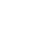# how to calculate the CAGR- Formula, Example and Calculator – A Comprehensive Guide

Contents

## how to calculate the CAGR: Theory

Calculating the Compound Annual Growth Rate (CAGR) can provide valuable insight into the performance of an investment or business over a given period of time. CAGR represents the rate at which an investment or business has grown on an annual basis, taking into account the effect of compounding. It is a useful metric for comparing the growth of different investments or businesses, as it eliminates the impact of the initial investment amount and the length of the investment period.

To calculate CAGR, you will need to know the beginning value of your investment or business (also known as the “initial value”), the ending value at the end of the investment period (also known as the “final value”), and the length of the investment period (in years).

## how to calculate the CAGR: Formula

CAGR = (final value / initial value)^(1/n) – 1

Where “n” is the number of years in the investment period.

For example, let’s say you have an investment that had a starting value of \$100,000 and a final value of \$150,000 after 5 years. To calculate the CAGR for this investment, we plug the values into the formula as follows:

CAGR = (\$150,000 / \$100,000)^(1/5) – 1

CAGR = (1.5)^(1/5) – 1

CAGR = 1.096 – 1

CAGR = 0.096, or 9.6%

This means that the investment grew at a compound annual rate of 9.6% over the 5-year period.

It’s important to note that CAGR only provides an average growth rate over the investment period. It does not take into account the fluctuations in the value of the investment or business during the period. For example, if the value of the investment increased significantly in the first few years and then leveled off or declined in the later years, the CAGR would still be calculated based on the average growth rate over the entire period.

## how to calculate the CAGR: Points to Keep in Mind

There are a few key points to keep in mind when using CAGR:

• CAGR is most useful for comparing the growth of different investments or businesses over a similar time period.
• CAGR does not take into account the impact of dividends or other distributions on the growth of an investment.
• CAGR assumes that any changes in the value of the investment or business are reinvested at the same rate.
• CAGR is an annualized rate, so it may not accurately reflect the actual growth of an investment or business over a shorter time period.

## how to calculate the CAGR: Where used

CAGR is often used to compare the performance of different investments and to measure the effectiveness of investment strategies. It is a useful metric for evaluating the growth of investments that may have had different starting and ending values, or for comparing investments that have had different periods of growth.

CAGR is used in a variety of contexts, including financial analysis, portfolio management, and business planning. It is commonly used by investors, financial analysts, and business owners to evaluate the performance of investments, assess the growth potential of a company or industry, and make informed decisions about future investments or business strategies.

CAGR is used to Forecast growth. Any industry that has a significant CAGR growth rate (Double Figures) should be considered attractive for investment.

CAGR:

## Conclusion

In conclusion, CAGR is a useful metric for evaluating the growth of an investment or business over a given period of time. It provides a way to compare the performance of different investments or businesses by taking into account the effect of compounding and eliminating the impact of the initial investment amount and the length of the investment period. By using the formula for CAGR, you can easily calculate the compound annual growth rate of any investment or business.

For more solutions to Case Studies Strategy and Human Resources, follow our blog

Do reach out to me on Linkedin or Quora.